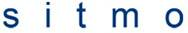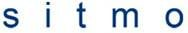# Angular Momentum Operator

leviathanX777
The operators used for the x and y components of angular momentum are:Show that Lx and Lz obey an uncertainty relation

2. No relevant equations.

## The Attempt at a Solution

I'm going on that the assumption that if LxLy - LyLz does not equal zero then they don't commute and have an uncertainty relation. However I can only get this equal to zero and don't know how to show the uncertainty rrelation if I achieve one.

## Answers and Replies

Plutoniummatt
I'm going on that the assumption that if LxLy - LyLz does not equal zero then they don't commute and have an uncertainty relation. However I can only get this equal to zero and don't know how to show the uncertainty rrelation if I achieve one.

if you mean:

[Lx, Ly] = LxLy - LyLx

then it does not equal to zero, angular moment is the cross product: r x p

so Lx = y.Pz - z.Py Ly = x.Pz - z.Px

where x and y and z are position operators and Px, Py and Pz are momentum operators, stick those into your commutator and try again, you should end up with

[Lx, Ly] = ihLz

where h is the reduced planck constant. and Lz is the Angular momentum operator for z axis

Staff Emeritus